How to Apply Principles of Capacitors and Inductors to a Communications Circuit

## How to Apply Principles of Capacitors and Inductors to a Communications Circuit

Key Terms

o         Antenna

o         Carrier wave

Objectives

o         Apply principles of capacitors and inductors to a simple communications circuit

o         Recognize the role of antennas in wireless communications

Although computers may be the most important outworking of electronics in the modern world, wireless communications isn't far behind. You may have wondered, for instance, how your cell phone or GPS device can send or receive information from distant sources--all invisibly.

Please note: do not attempt to replicate the diagrams, illustrations, or instructions in this article in a real-life scenario. Doing so may result in electric shock, injury, or death. These examples are provided only for theoretical discussion and not for actual/physical use.

Transformers are in some sense an example of wireless communications: one circuit "communicates" with another without any physical (conducting) connection. In effect, each inductor acts as an antenna, which transmits and/or receives an electromagnetic signal.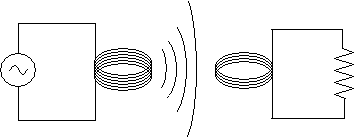Although this situation is not precisely analogous to, for example, radio communications (cell phones, walkie talkies, and so on), it is very close.

Antennas

You've probably seen a number of different antennas, although you may not have recognized them as such. They come in any number of shapes and sizes, such as the "whip" antenna on your car (also called a monopole antenna), a horn antenna on a radio tower, and a dish antenna (for receiving satellite television). In a circuit diagram, antennas are often represented using the symbol below.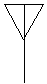When a signal (a varying voltage or current) is supplied to an antenna, the electrons in the antenna move back and forth in response, creating a variation in the electromagnetic field that travels in various directions (depending on the configuration of the antenna). Other antennas can detect this variation--the changing field creates a current in the receiving antenna, which can then be amplified into a signal that is fed to your radio or television, for instance.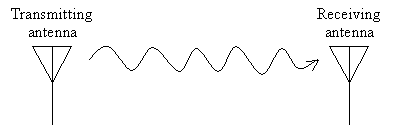Picking a Frequency

Modern radios are fairly complicated, and they involve a combination of simple electrical components (resistors, for instance) as well as semiconductor devices. To illustrate how we can "tune" to a specific frequency, we can look just at concepts we have studied thus far: specifically, circuits that use inductors and capacitors.

Recall that a capacitor stores energy by "gathering" charge (effectively, it is storing energy in the electric field). It can discharge this energy by creating a current from one plate to another. An inductor, on the other hand, stores energy in the magnetic field when current flows through it. The inductor "discharges" when the current falls.

But what happens if we put a capacitor and inductor in a circuit together?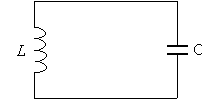Assume the capacitor is charged; it will begin to discharge by sending a current through the inductor.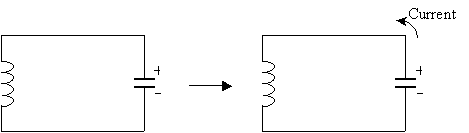But the inductor converts some of this current to magnetic field energy. The capacitor will continue to supply current until it is discharged, but once this happens, the inductor will convert its magnetic field back into current, effectively recharging the capacitor (but in reverse relative to its original charge, as shown below).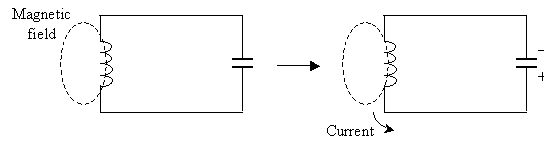Eventually, the magnetic field is depleted, but the capacitor has been recharged!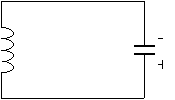The process then repeats, but this time in the opposite direction. The frequency (number of "cycles" per second) for this process is determined by the capacitance of the capacitor and the inductance of the inductor. In effect, this process is an electrical equivalent of a spring: energy simply is passed back and forth between the magnetic field (the inductor) and the electric field (the capacitor). Because all of these components, in reality, have some resistance, eventually the process will come to a halt when all the energy is converted to heat (i.e., it is dissipated by the resistance of the components, including the wires).

This phenomenon can be used to "tune" a circuit to a particular carrier wave. Let's say we connect this circuit to an antenna as shown below. Notice, first, that we use the following circuit symbol to represent ground: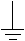Simply think of ground as the reference point for our voltage measurements: we simply define it as zero volts. Our simple tuning circuit is shown below on the left. (Note that it is essentially identical to a more familiar circuit, shown on the right.)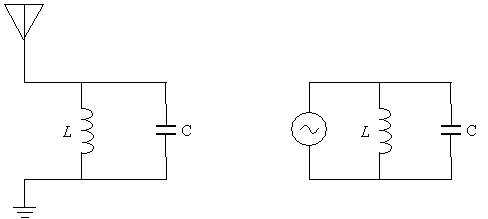This circuit will "pick out" the portion of the antenna signal at the frequency of the circuit described above and will reduce all other signal frequencies. When the inductance and capacitance are chosen correctly, the circuit would then be tuned for a particular radio station frequency, for instance.

Of course, there is much more to a radio than just this type of tuning circuit (this is actually a simplistic approach, but it represents the concepts). The signal must then be amplified and "cleaned up" before it is sent to a speaker, which converts the electrical signal to sound (following our example of a radio). But this simple circuit shows how, using the ability to transmit signals using electromagnetic waves, we can use electronics to pick a particular signal out of many competing signals at different frequencies.

In many cases, we have only been able to very briefly introduce concepts, like radio communications, semiconductor devices, antennas, and so on. A thorough understanding of these areas requires complicated mathematics and more-detailed concepts. Of course, ultimately, large and complicated circuits are too much to fully understand using simple techniques; thus, many circuits are designed and analyzed using computer programs. But by understanding the qualitative principles of electronics, you will have a foundation to study broader and more-specific areas in this field.

Explore